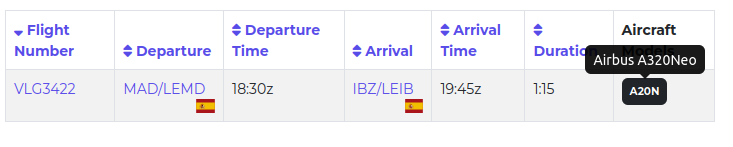In engineering, specially in the FPGA field, there are several sensitive fields because of their consequences in case of failure. Those fields involve defense, space, cyber security or aviation. In in this last field where we are going to navigate deeply, more precisely in one of its communication system, the Mode Select Beacon System (MODE S). This technology allow the aircraft share its position, speed or altitude in broadcast mode, so any receiver device can receive that information and obtain the aircraft information. This technology is the reason because pages like flightradar24 exists. This pages have many MODE S receivers along the world receiving information about the different flights. In this post we are going to fly inside the MODE S frames, and I will show you how to receive and decode MODE S frames using the USRP B210 and MATLAB using the Communications Toolbox.

As a disclaimer, I have to say that I am not an expert in aviation, and the post will be focused on the reception, demodulating and decoding of this protocol.

Aircraft surveillance is very important nowadays becaus of the huge number of flights that are crossing out countries, and, although we can think that the size of the air space is almost infinity, the true is that exists different highways where all the flights, according its origin and destination navigate, so know the position and the speed of all the aircraft turns very important. Throughout the aviation history, there was different methods to know the aircraft position. Primary Surveillance Radar (PSR) was the first method, and it was based on sending of a 1 us pulse from the control tower, and measure the time that the signal spends to reach the aircraft, and be reflected and reveiced again by the antenna. A second evolution was the Secondary Surveillance Radar (SSR). This uses the PSR and using a different frequency, the control tower was able to receive information about the aircraft. In 1990 a new evolution in aircraft surveillance was introduced, the Mode Select Beacon System (MODE S), whish is used today as the main sources for aircraft surveillance. In MODE S, and traffic controller sends a request (uplink) to the aircaft, and the the aircraft responds (downlink) with the requested information.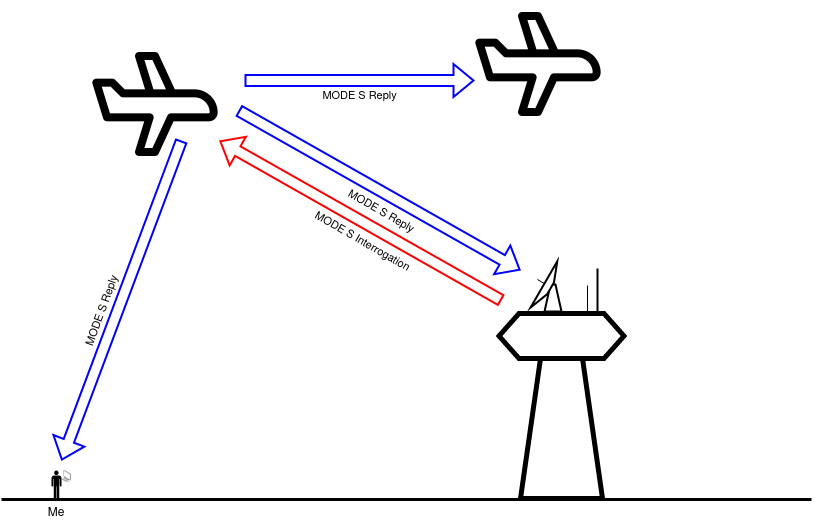The uplink frame is sent using a 1030 Mhz carrier and modulated using phase modulation (PSK), unlike the downlink format which is sent using a 1090 MHz carrier and uses amplitude modulation (ASK).

Several years later ADS-B appeared. This technology includes the word automatic, so there is no input needed from the pilot or the controller, the system sends periodically information about altitude, position of speed. Each ADS-B sending is itself contained, so we can obtain the information using only the frame received.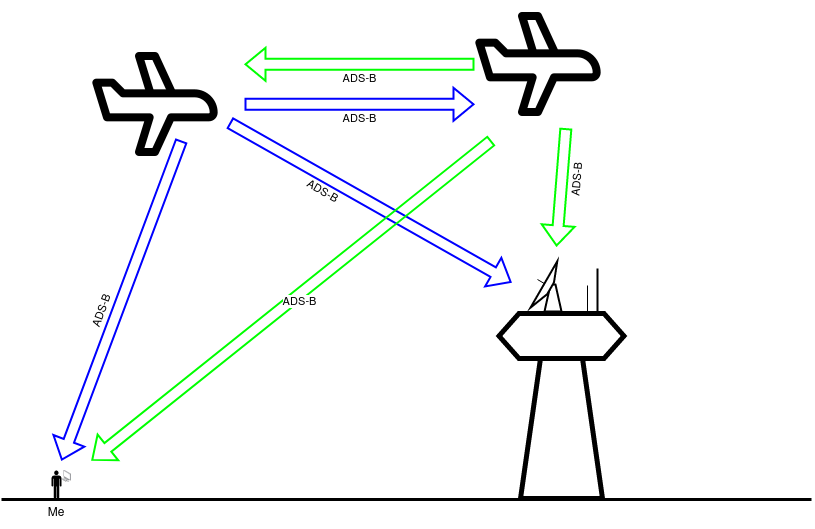ADS-B, like MODE S downlink format, uses 1090 MHz as a carrier and it is modulated using ASK modulation.

In this post we are going to focus in Mode S frames. The entire ModeS reply frame is the next. We will have a preamble of eight bits, and then a data frame that could have 56 or 112 bits. it is important to know that Mode S data is codified using Manchester encoding, so a logic one is encoded as a falling edge (1 - 0), and a logic zero is encoded as a rising edge (0 - 1).On the data frame we can find the next fields.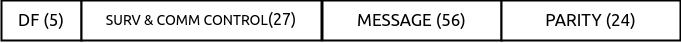According its DF code, we can find out the content of the message. Different DF codes are shonw in the next table.

DF Frame content
0 Short ACAS
4 Surveillance altitude
5 Surveillance IDENT
16 Long ACAS
17 Extended squitter

At this point, we already know the modulation method, and the carrier, so we can start to receive this signal to check how they looks. As I mentioned before, we are going to use the USRP B210, and MATLAB to first, receive the signal in Simulink, and second, decode the signal with an script.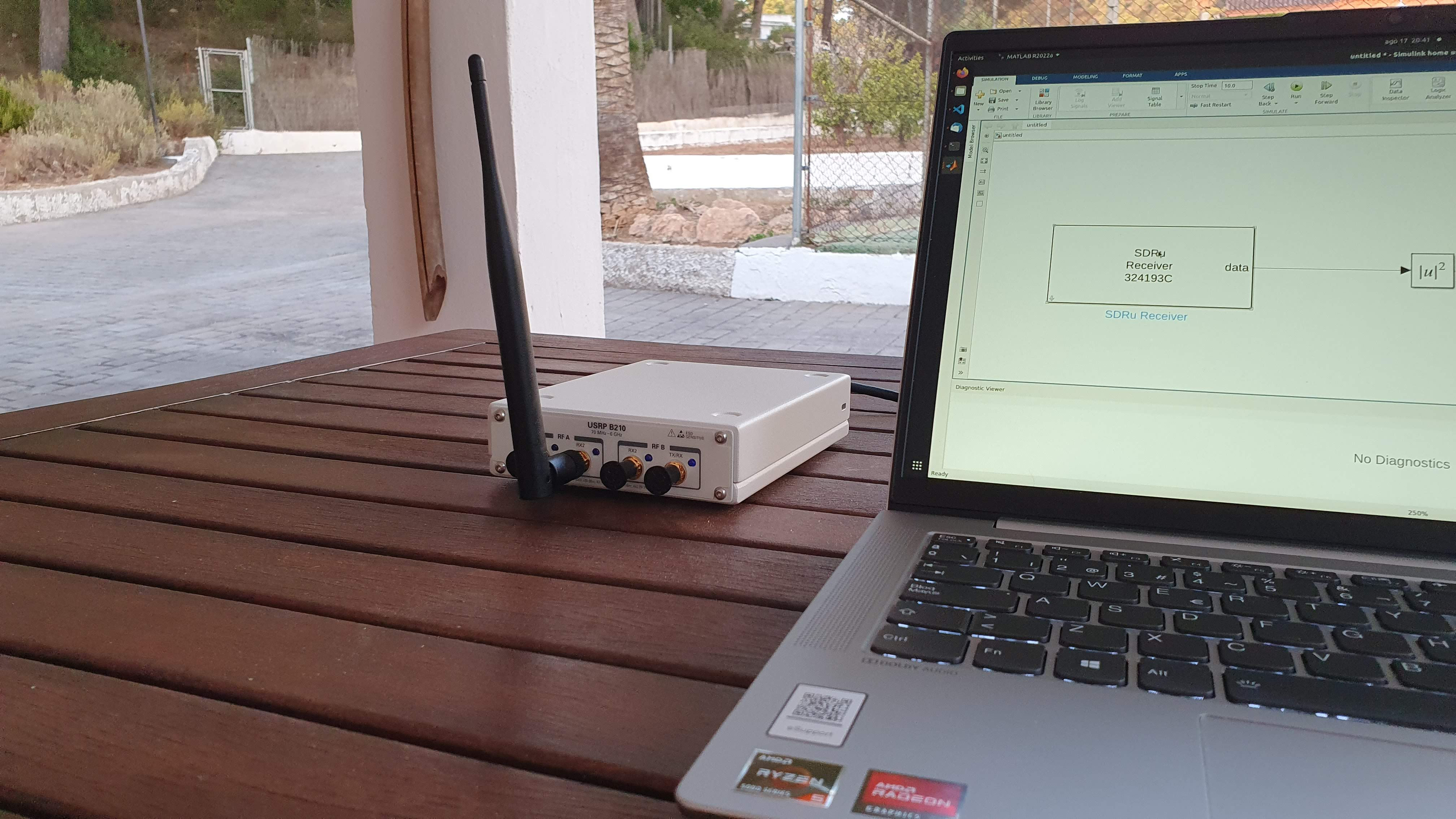In order to connect the USRP B210 to Simulink, we have to install the hardware support package for USRP devices. Once the USRP is HSP is installed, in Simulink we will see a new library with 2 different blocks, the first one for use the USRP as a receiver, and the second one to use the USR as a transmitter.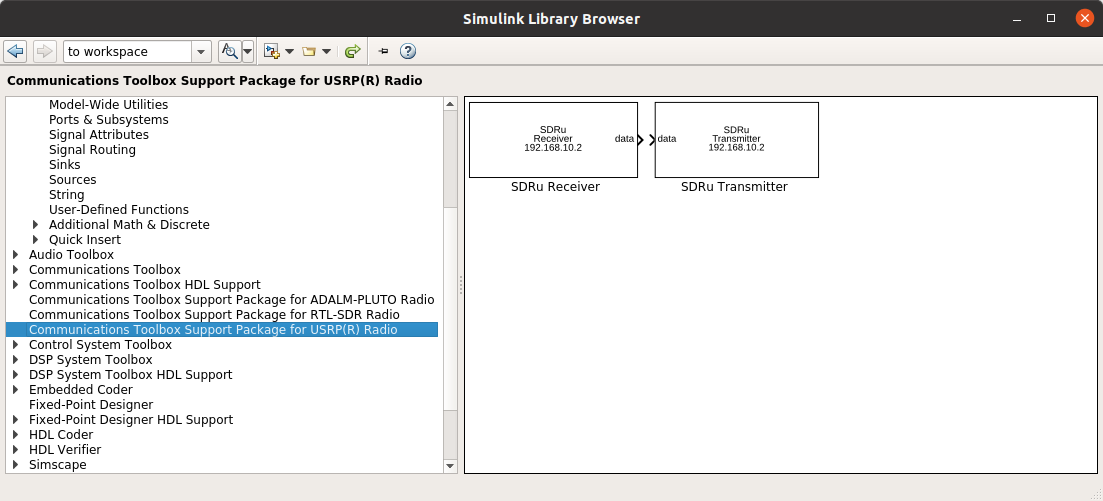For this post, we are going to use the USRP to receive a MODE S signal, so we have to add to the model the receiver block.The configuration of the SDRu block is the next.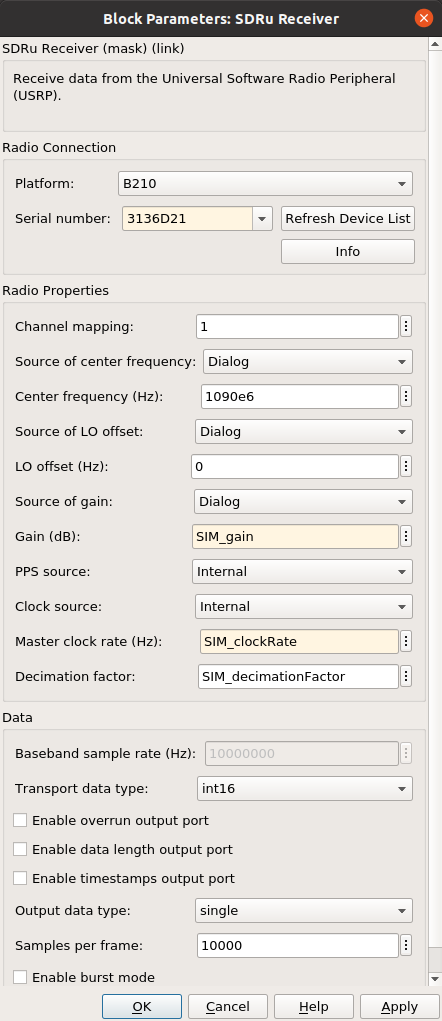In order to be able to change the configuration of the USRP using an script, all the radio parameters are configured using variables.

% SDRu configuration

SIM_gain = 64;
SIM_clockRate = 10e6;
SIM_decimationFactor = 1;


The output format of the receiver block is configured using the parameters inside the group data. In order to configure these parameters, first we need to know the output format of the block, which is the data received from the USRP B210. The demodulation that USRP execute is a complex demodulation. This means that the signal received is demodulated using 2 different signals, $$cos(fc)$$, and $$sin(fc)$$, the output of the cosine demodulation is the real part, and the output of the sine demodulation is the imaginary part. This kind of demodulation is very useful when we need demodulate the signal using more complex demodulations, but in this case, the demodulation of an ASK, we only need to know the module of the vector defined by the imaginary and the real part. In addition to the numeric format of the output, since data is acquired at very high rate, 10 Msps in our case, the receiver block has a internal cache, to receive data at high rate, and then, once the cache is filled with a configured number of samples, the output of the module will return a vector with all the samples, then the output is deactivated until the cache is filled again.

Regarding the configuration, we can configure the Transport data type, which is the format of the data that comes from the USRP. Remember that USB is a serial protocol, so according the width of the data, the speed will change. In this case we can select int16 or int8. Configuring int8 we can double the speed than using int16. On the other hand, the data using int8 is quantized using only 8 bits instead of 16. Besides this configuration, the block performs a transformation to a floating number again, and the parameter Output data type will configure the precision of the output data. Finally the parameter Samples per frame will configure the size of the internal cache.

Once we have the SDRu block on the model, we can complete the model with a few more blocks. Since we need to know the value of the module of the signal, we have to add Magnitude square, thus the output of this block will be a real number. Also, since the magnitude is squared, it will help to increase the amplitude of the signal when it is greater than zero. Then, following this block, we are going to add an Unbuffer block. This block will serialize the data that receiver block returns in blocks of 10k samples. In this way we will have a single vector with $$f_s \cdot time$$ samples. Finally, since all the processing will be executed in a MATLAB script, we need to add a To workspace block to send data to the workspace. The resulting Simulink diagram is the next.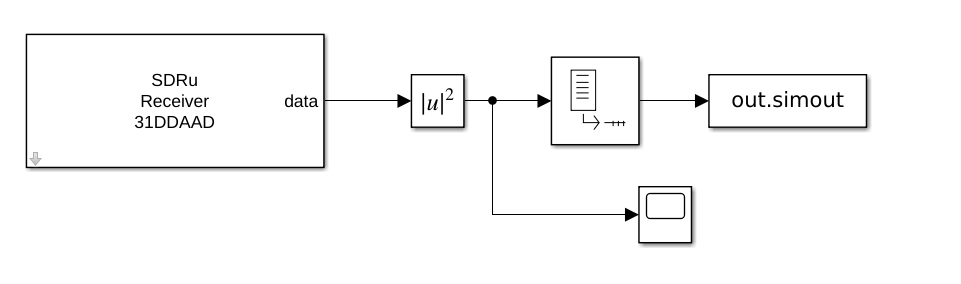Once the simulink diagram is complete, we can run it, and data acquired by the USRP will be sent to the workspace. In the next figure we can see how looks the data captured in 0.5s with the above configuration. We can see how different frames are captured, and how they can be separate from the noise level of the signal.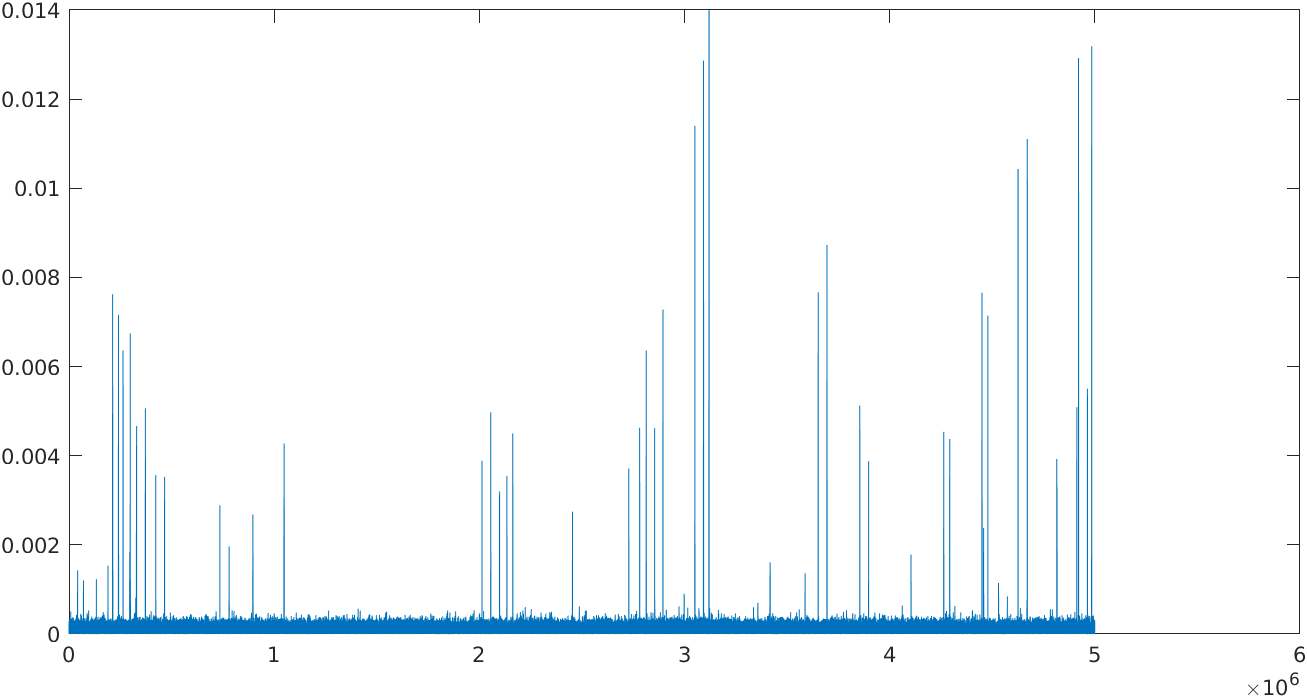If we apply zoon to one of this frames, we can see the binary data modulated using ASK. The amplitude of the ones will depend of the power received. This power depends of the gain of the antenna, the power of the emitter and the distance where the aircraft is sending the signal. This amplitude can be increased by increasing the gain using the SIM_gain variable.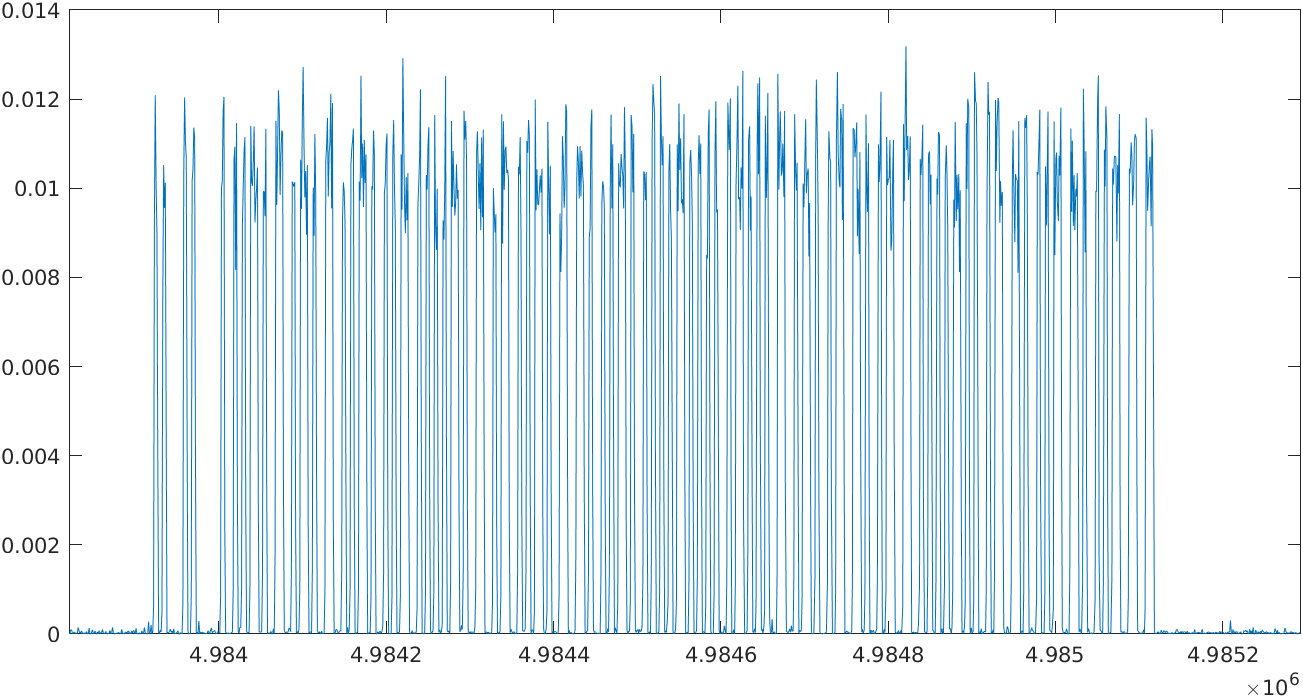To extract the binary data, I have configured a threshold. Then, if the signal is greater than the threshold, it means a logic ‘1’, by contrast, if the signal is smaller than the threshold, it means a logic zero. A simple MATLAB script to extract this data is the next.

function ModeS_data = ModeS_oneFrameBinary (usrp_data, data_length, offset)

ModeS_threshold = 0.0005;
temp_data = [];

ModeS_data = [zeros(1, data_length)];

data_vector = squeeze(usrp_data);

size(data_vector)
for i = offset:offset+data_length
if (data_vector(i) > ModeS_threshold)
temp_data = [temp_data, 1];
else
temp_data = [temp_data, 0];
end
end

ModeS_data = temp_data;

figure
plot(data_vector(offset:offset+data_length) * 100/1.2)
hold on
plot(temp_data, '-*')

end


Using this script the result is the next figure.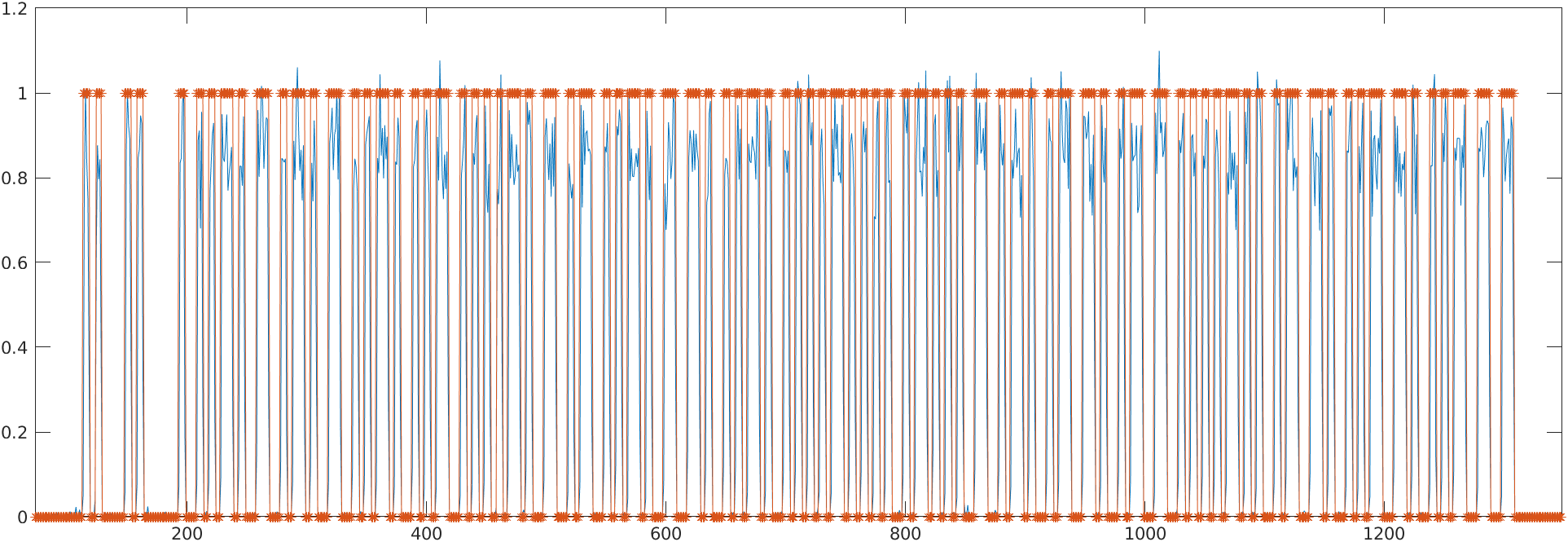We can see the preamble that is a fixed binary string 10100001010000, and then the data, that is different on each frame.

Since frame is oversampled ten times, for each bit we have 10 samples. Using the function reshape, we can split the entire data frame in groups of ten bits, and we can see the manchester encoding.


>> ModeS_dataBits = reshape(frame,10,[]).'

ModeS_dataBits =

1     1     1     1     1     0     0     0     0     1
1     1     1     1     1     0     0     0     0     0
0     0     0     0     0     0     0     0     0     0
0     0     0     0     1     1     1     1     1     1
0     0     0     0     1     1     1     1     1     1
0     0     0     0     0     0     0     0     0     0
0     0     0     0     0     0     0     0     0     0
0     0     0     0     0     0     0     0     0     0
0     0     0     0     1     1     1     1     1     1
1     1     1     1     1     0     0     0     0     0
0     0     0     0     1     1     1     1     1     1
1     1     1     1     1     0     0     0     0     1
1     1     1     1     1     0     0     0     0     1
1     1     1     1     1     0     0     0     0     0
0     0     0     0     1     1     1     1     1     1
1     1     1     1     1     0     0     0     0     0
0     0     0     0     1     1     1     1     1     1
0     0     0     0     1     1     1     1     1     1
1     1     1     1     1     0     0     0     0     1
1     1     1     1     1     0     0     0     0     0
0     0     0     0     1     1     1     1     1     1
1     1     1     1     1     0     0     0     0     0
...


In order to decode the frame into an hexadecimal data, we need first to decode the binary data encoded using manchester encoding, and then convert the binary data to hexadecimal data. To decode the manchester codification, we can read each bit in the first quarter, that for a sampling frequency of 10 Msps, will be in the sample 2.5. Then we have to convert into hexadecimal each group of 4 bits. The MATLAB code that execute these actions is the next.

function hex_frame = ModeS_decodeBits (data, SIM_clockRate, SIM_decimationFactor)

% split data un groups
ModeS_dataBits = reshape(data,10,[]).';

% data decoding excluding preamble
ModeS_databin = [];

% obtain the samples where we have to read
ModeS_SamplesPerBit_Preamble= floor(SIM_clockRate / SIM_decimationFactor / 2e6);
samplesMiddleBitStart = floor((ModeS_SamplesPerBit_Preamble+0.5)/2);

% read all bits excluding preamble
for i = 9:120
ModeS_databin = [ModeS_databin, int2str(ModeS_dataBits(i,samplesMiddleBitStart))];
end

hex_frame = dec2hex(bin2dec(reshape(ModeS_databin,4,[]).')).';

end


The result of this script, passing as argument the variable frame which is the output of the ModeS_oneFrameBinary function is the next.

>> ModeS_dataHex = ModeS2hex(frame, SIM_clockRate, SIM_decimationFactor)

ModeS_dataHex =

'8D34620D2358C1F3D32CA0E58B15'


Then, once the Mode S data is decoded, we can use the hexadecimal frame with the Python package pyModeS and decode the Mode S message.

import pyModeS as pms

pms.tell("8D34620D2358C1F3D32CA0E58B15")

Message: 8D34620D2358C1F3D32CA0E58B15